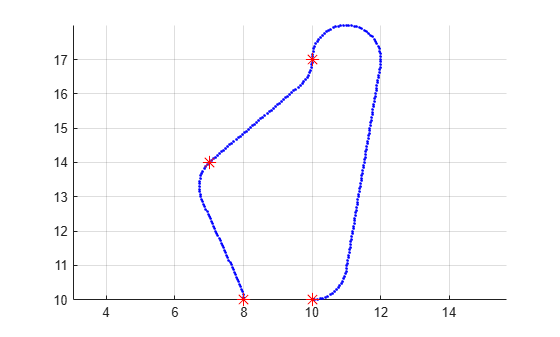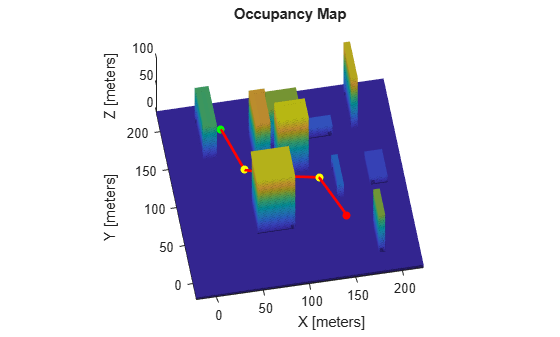# navPath

Planned path

Since R2019b

## Description

The `navPath` object stores paths that are typically created by geometric path planners. Path points are stored as states in an associated state space.

## Creation

### Syntax

``path = navPath``
``path = navPath(space)``
``path = navPath(space,states)``
``path = navPath(space,states,maxNumStates)``

### Description

````path = navPath` creates a path object, `path`, using the SE(2) state space with default settings.```

example

````path = navPath(space)` creates a path object with state space specified by `space`. The `space` input also sets the value of the StateSpace property.```
````path = navPath(space,states)` allows you to initialize the path with state samples given by `states`. Specify `states` as a matrix of state samples. States that are outside of the `StateBounds` of the state `space` object are reduced to the bounds. The `states` input also sets the value of the States property.```
````path = navPath(space,states,maxNumStates)` creates a path object with the specified maximum number of states allowed in path `maxNumStates`. The `maxNumStates` input also sets the value of the MaxNumStates property.```

## Properties

expand all

State space for the path, specified as a state space object. Each state in the path is a sample from the specified state space. You can use objects such as `stateSpaceSE2`, `stateSpaceDubins`, `stateSpaceReedsShepp`, or `stateSpaceSE3` as a state space object. You can also customize a state space object using the `nav.StateSpace` object.

States of the path, specified as a real-valued M-by-N matrix. M is the number of states in the path, and N is the dimension of each state. You can only set this property during object creation or using the `append` function.

Example: `[0 0 0; 1 1 0; 2 2 0]`

Example: ```[0 0 0 1 0 0 0; 1 1 1 1 0 0 0; 2 2 1 1 0 0 0]```

Data Types: `double`

Number of state samples in the path, specified as a nonnegative integer. The number is the same as the number of rows of the state matrix specified in the States property.

Data Types: `double`

Maximum number of states allowed in the path, specified as a positive scalar integer.

When specified as `inf`, the path is explicitly resizeable. For code generation, `DynamicMemoryAllocation` must be set to `'On'`.

When specified as a positive scalar integer, the maximum number of states in the object is limited to the specified value. Use this to create a resizeable path when enabling `DynamicMemoryAllocation` is not allowed during code generation.

Data Types: `double`

## Object Functions

 `append` Add states to end of path `copy` Create copy of path object `interpolate` Interpolate points along path `pathLength` Length of path

## Examples

collapse all

Create a `navPath` object based on multiple waypoints in a Dubins space.

`dubinsSpace = stateSpaceDubins([0 25; 0 25; -pi pi])`
```dubinsSpace = stateSpaceDubins with properties: SE2 Properties Name: 'SE2 Dubins' StateBounds: [3x2 double] NumStateVariables: 3 Dubins Vehicle Properties MinTurningRadius: 1 ```
`pathobj = navPath(dubinsSpace)`
```pathobj = navPath with properties: StateSpace: [1x1 stateSpaceDubins] States: [0x3 double] NumStates: 0 MaxNumStates: Inf ```
```waypoints = [8 10 pi/2; 7 14 pi/4; 10 17 pi/2; 10 10 -pi]; append(pathobj,waypoints)```

Interpolate that path so that it contains exactly 250 points.

`interpolate(pathobj,250)`

Visualize the interpolated path and the original waypoints.

```figure grid on axis equal hold on plot(pathobj.States(:,1),pathobj.States(:,2),".b") plot(waypoints(:,1),waypoints(:,2),"*r","MarkerSize",10)```Calculate length of path.

```len = pathLength(pathobj); disp("Path length = " + num2str(len))```
```Path length = 19.4722 ```

Load a 3-D occupancy map of a city block into the workspace. Specify the threshold to consider cells as obstacle-free.

```mapData = load("dMapCityBlock.mat"); omap = mapData.omap; omap.FreeThreshold = 0.5;```

Inflate the occupancy map to add a buffer zone for safe operation around the obstacles.

`inflate(omap,1)`

Create an SE(3) state space object with bounds for state variables.

`ss = stateSpaceSE3([0 220;0 220;0 100;inf inf;inf inf;inf inf;inf inf]);`

Create a `navPath` object based on multiple waypoints in an SE(3) state space.

```path = navPath(ss); waypoints = [40 180 15 0.7 0.2 0 0.1; 55 120 20 0.6 0.2 0 0.1; 100 100 25 0.5 0.2 0 0.1; 130 90 30 0.4 0 0.1 0.6; 150 33 35 0.3 0 0.1 0.6]; append(path,waypoints)```

Interpolate that path so that it contains exactly 250 points.

`interpolate(path,250)`

Visualize the interpolated path and the original waypoints.

```show(omap) axis equal view([-10 55]) hold on % Start state scatter3(waypoints(1,1),waypoints(1,2),waypoints(1,3),"g","filled") % Goal state scatter3(waypoints(end,1),waypoints(end,2),waypoints(end,3),"r","filled") % Intermediate waypoints scatter3(waypoints(2:end-1,1),waypoints(2:end-1,2), ... waypoints(2:end-1,3),"y","filled") % Path plot3(path.States(:,1),path.States(:,2),path.States(:,3), ... "r-",LineWidth=2)```Calculate length of path.

```len = pathLength(path); disp("Path length = " + num2str(len))```
```Path length = 204.1797 ```

## Version History

Introduced in R2019b